### 2. Abstract

Convolutional layers are the core building blocks of Convolutional Neural Networks (CNNs).

In this paper, we propose to augment a convolutional layer with an additional depthwise convolution, where each input channel is convolved with a diﬀerent 2D kernel. The composition of the two convolutions constitutes an over-parameterization, since it adds learnable parameters, while the resulting linear operation can be expressed by a single convolution layer.

We refer to this depthwise over-parameterized convolutional layer as DO-Conv.

• 卷积层是卷积神经网络(CNNs)的核心构件。
• 在本文中，我们提出通过附加的depthwise卷积来增强卷积层，其中每个输入通道都使用不同的2D kernel进行卷积。两个卷积的组成构成了over-parameterization，因为它增加了可学习的参数，而生成的线性运算可以由单个卷积层表示
• 我们将此depthwise over-parameterized卷积层称为DO-Conv。

• 我们通过大量实验表明，仅用DO-Conv层替换常规卷积层就可以提高CNN在许多经典视觉任务（例如图像分类，检测和分割）上的性能。

• 此外，在推理阶段，深度卷积被折叠为常规卷积，从而使计算量精确地等于卷积层的计算量，而没有over-parameterization。由于DO-Conv引入了性能提升，而不会导致推理的计算复杂性增加，因此我们提倡将其作为传统卷积层的替代方法。

### 3. Introduction

• 论文首先指出了over-parameterization的优势

It has been widely accepted that increasing the depth of a network by adding linear and non-linear layers together can increase the network’s expressiveness and boost its performance.

On the other hand, adding extra linear layers only is not as commonly considered, especially when the additional linear layers result in an over-parameterization 1 — a case where the composition of consecutive linear layers may be represented by a single linear layer with fewer learnable parameters.

Though over-parameterization does not improve the expressiveness of a network, it has been proven as means of accelerating the training of deep linear networks, and shown empirically to speedup the training of deep non-linear networks

• 指出本文提出的新的卷积构造思路

In this work, we propose to over-parameterize a convolutional layer by augmenting it with an “extra” or “over-parameterizing” component: a depthwise convolution operation, which convolves separately each of the input channels.

We refer to this depthwise over-parameterized convolutional layer as DO-Conv, and show that it can not only accelerate the training of various CNNs, but also consistently boost the performance of the converged models.

• 通常，过度参数化的一个显着优势是，在训练阶段之后，可以将过度参数化所使用的多层复合线性运算折叠为紧凑的单层表示形式。 然后，在推理时仅使用单个层，从而将计算减少到与常规层完全等效。

One notable advantage of over-parameterization, in general, is that the multi-layer composite linear operations used by the over-parameterization can be folded into a compact single layer representation after the training phase. Then, only a single layer is used at inference time, reducing the computation to be exactly equivalent to a conventional layer.

### 4. Method

#### 4.1 Notation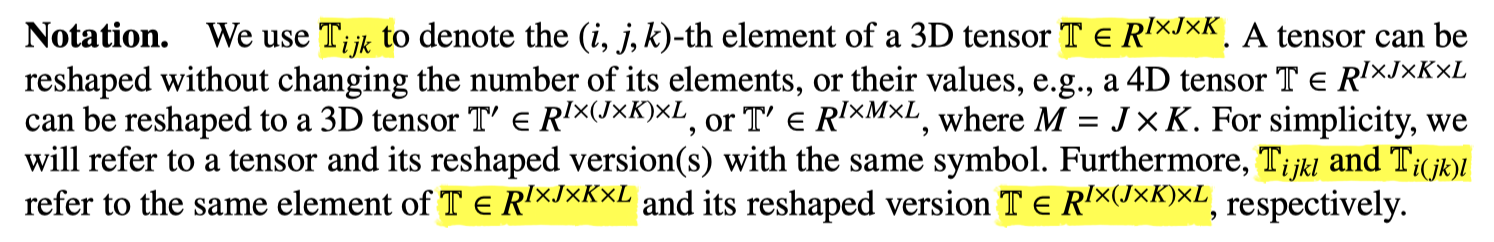• 上述论文介绍了使用的几个Tensor的代表符号

#### 4.2 Conventional convolutional layer

• 此部分的标题为传统的卷积层，介绍了常规卷积层的操作，以下为论文原文部分：
• 论文给出的示意图和解释有些抽象，W为卷积核，P为输入，其中，M x N代表了之前的大小，现在将其合并，将抽象的通道维度单独表示了出来；
• 可参考之前的笔记：深度可分离卷积和普通卷积的区别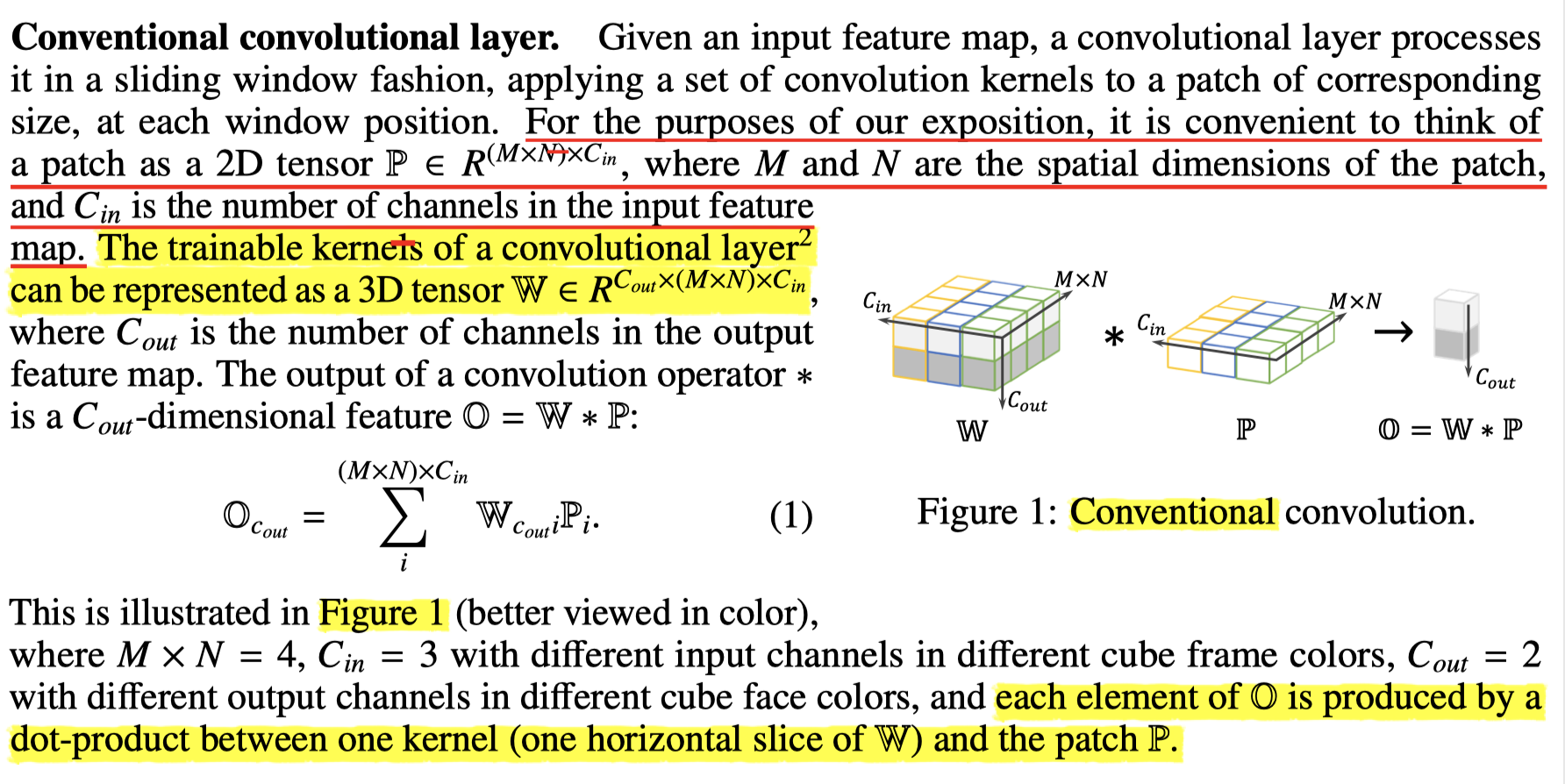#### 4.3 Depthwise convolutional layer

• 参考理解：之前写过的一篇深度可分离卷积和普通卷积的区别
• W的D_mul相当于卷积的个数，分离卷积是每个通道进行一次卷积，因为卷积核的每个通道的维度为M x N，所以每次卷积只有一个数值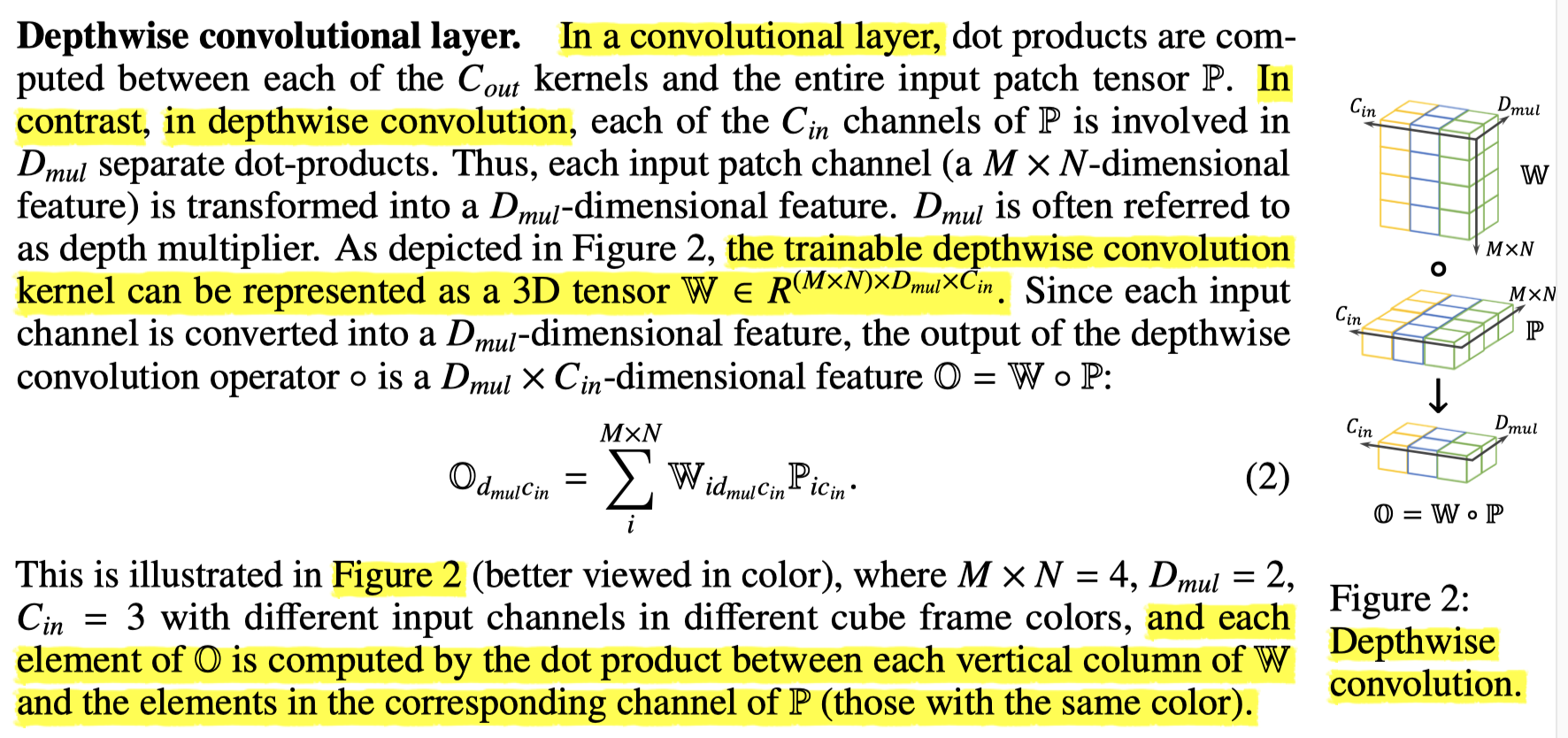#### 4.4 Depthwise over-parameterized convolutional layer (DO-Conv)

• 论文提出的DO-Conv是前面两种卷积方式的组合，具体分为feature-composition和kernel-composition，论文给出了配图+解释如下：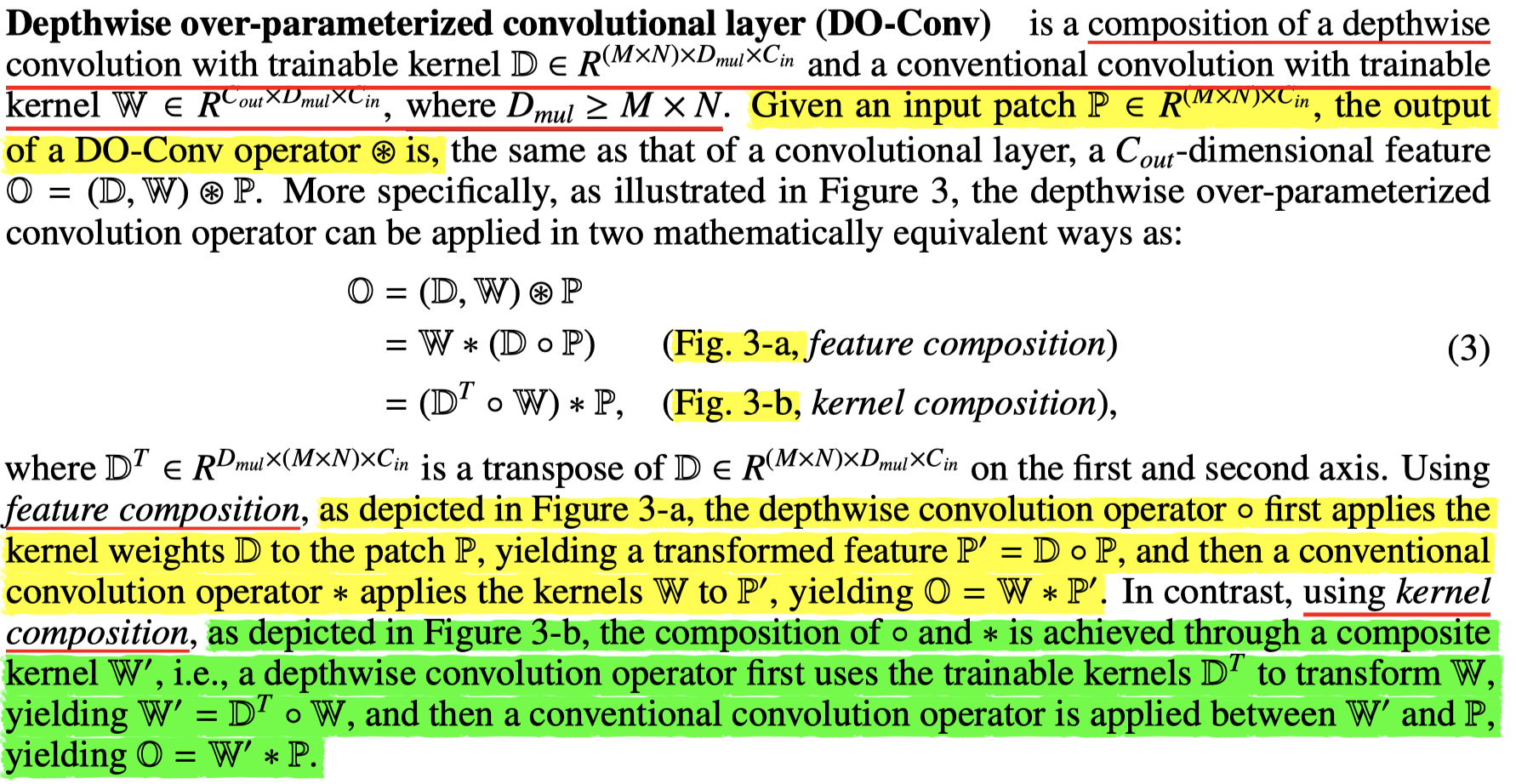• 图示如下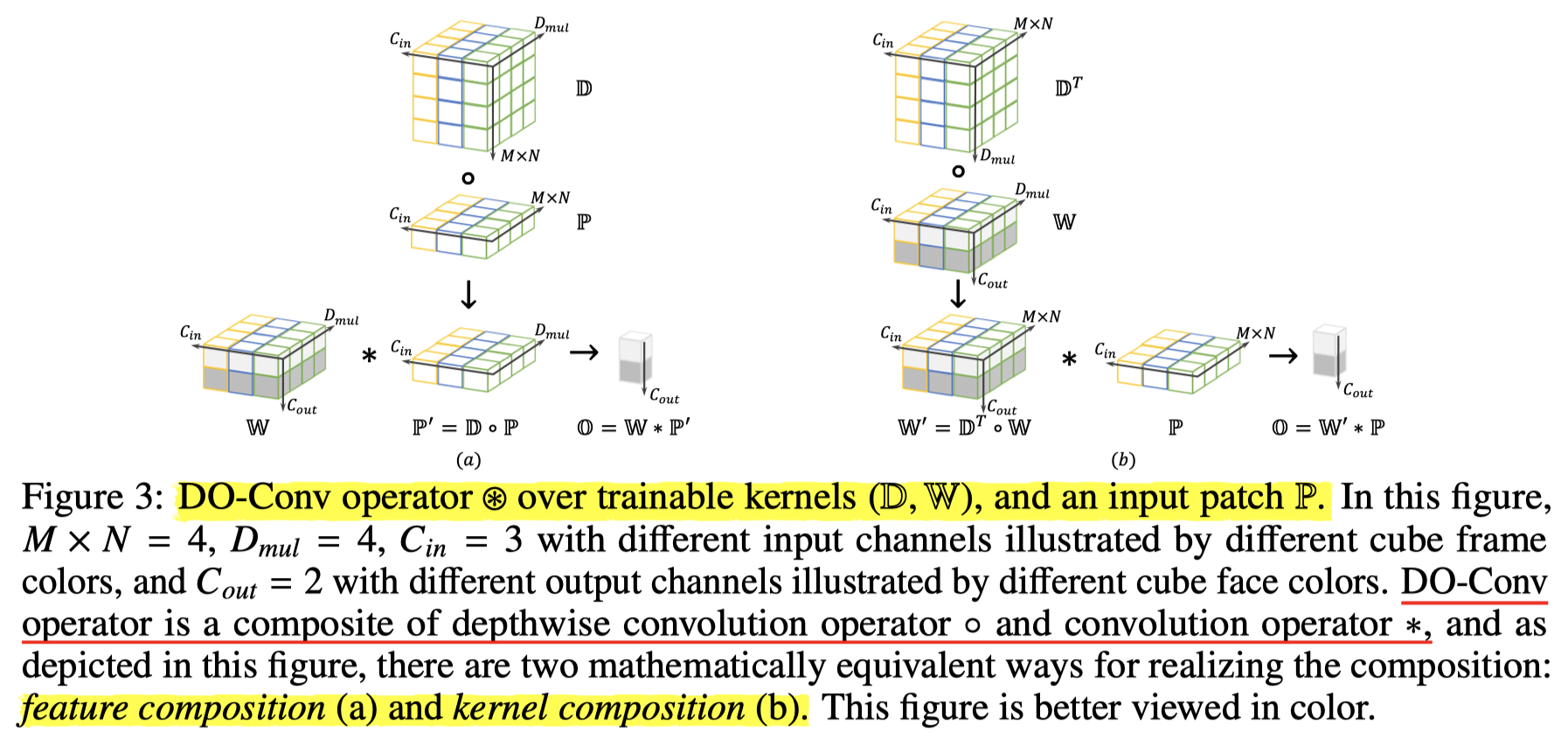#### 4.5 DO-Conv is an over-parameterization of convolutional layer

• 论文此部分解释了DO-Conv是过参数层的原因
• 最后波浪线的部分不理解，表达了D_mul必须大的原因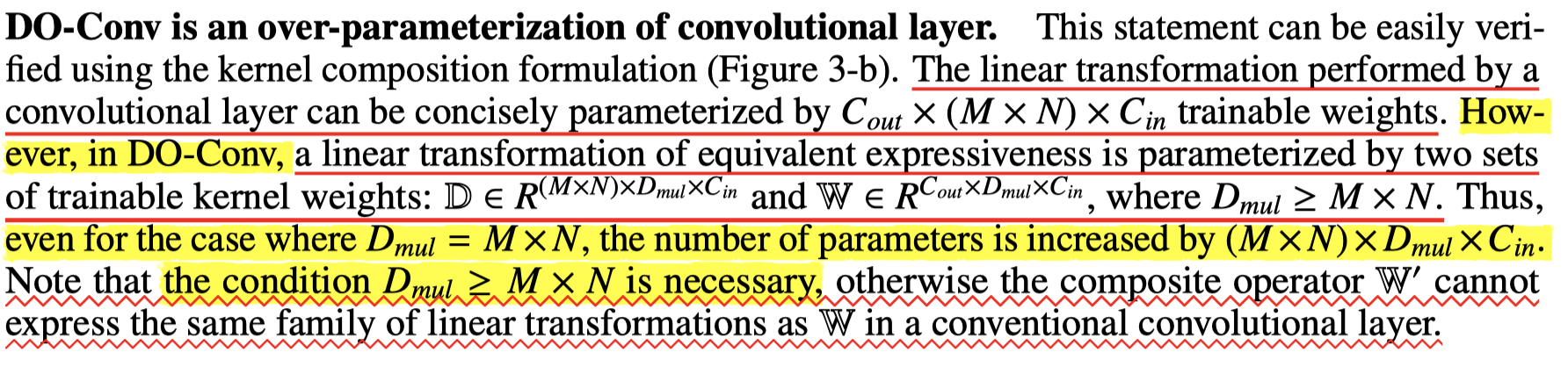#### 4.6 Training and inference of CNNs with DO-Conv.

• 此部分稍微提了一下训练和验证阶段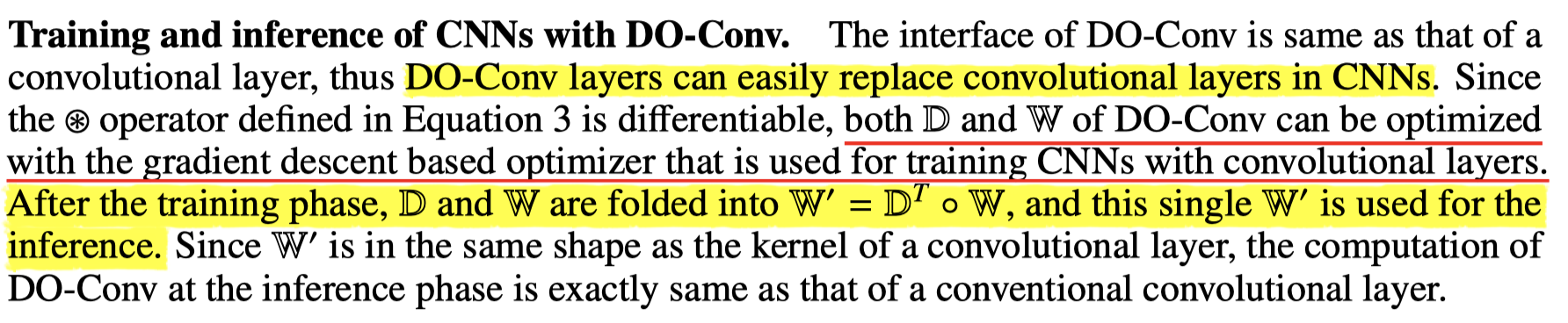#### 4.7 Training eﬃciency and composition choice of DO-Conv

• 计算了两种组合方式的训练效率，指出了kernel composition 更适合训练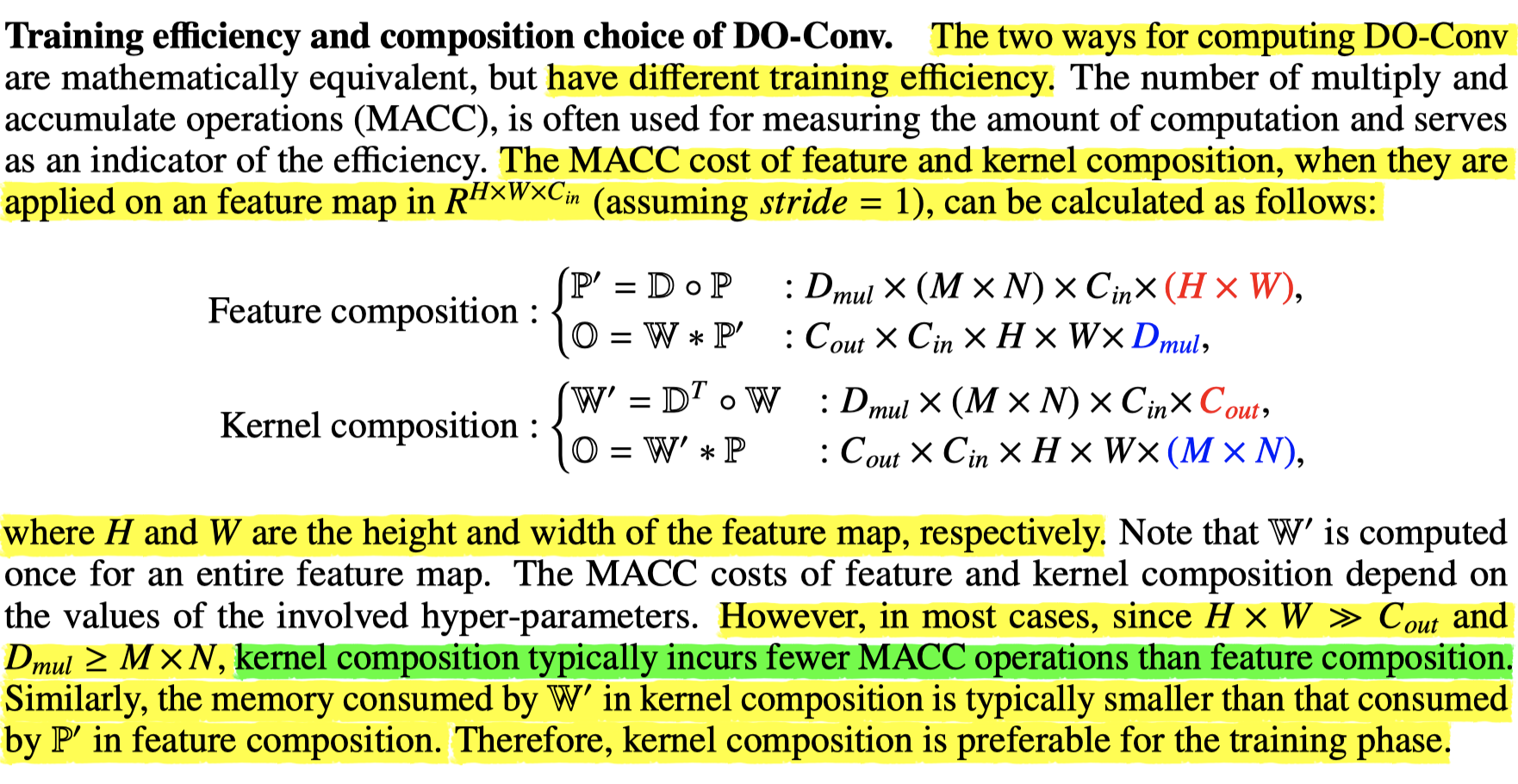#### 4.8 DO-Conv and depthwise separable convolutional layer

• 此部分讲解了DO-Conv和深度分离卷积两者的相似和区别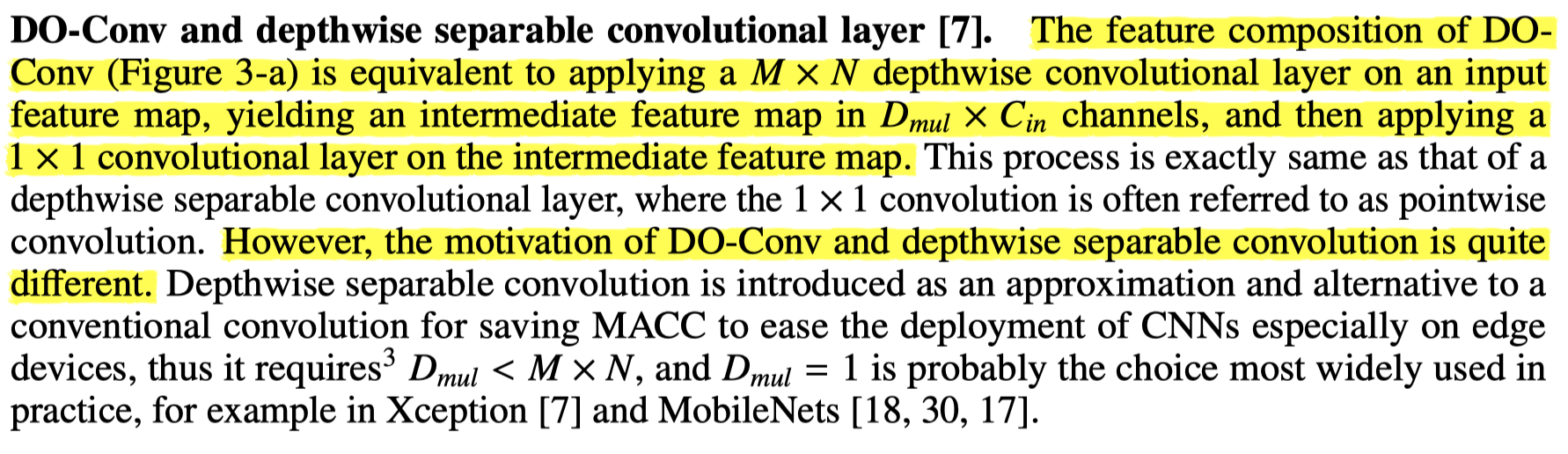#### 4.9 Depthwise over-parameterized depthwise/group convolutional layer (DO-DConv/DO-GConv)

• 介绍了只使用深度分离卷积进行组合而得到的DO-DConv/DO-GConv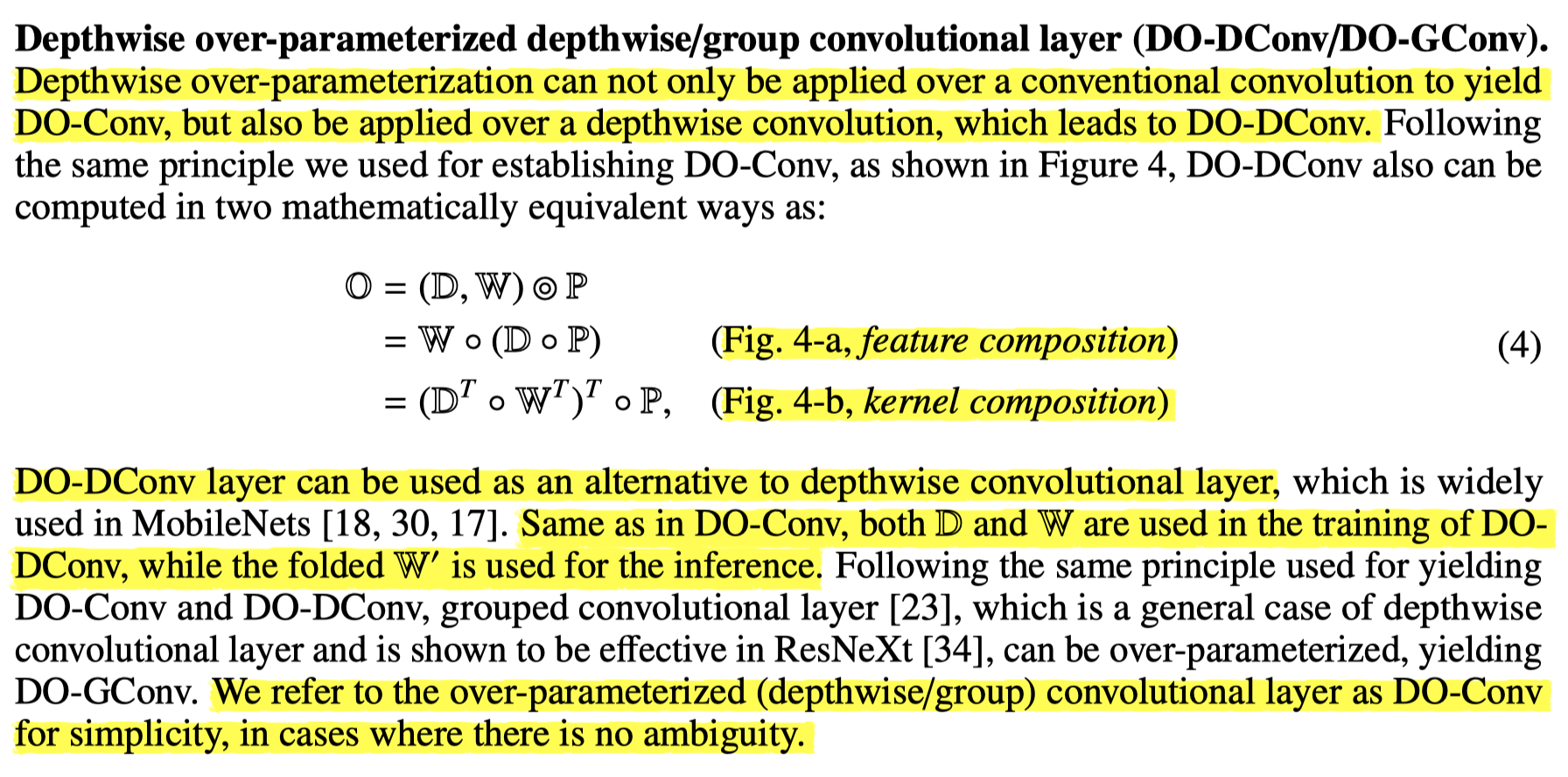• 图示如下：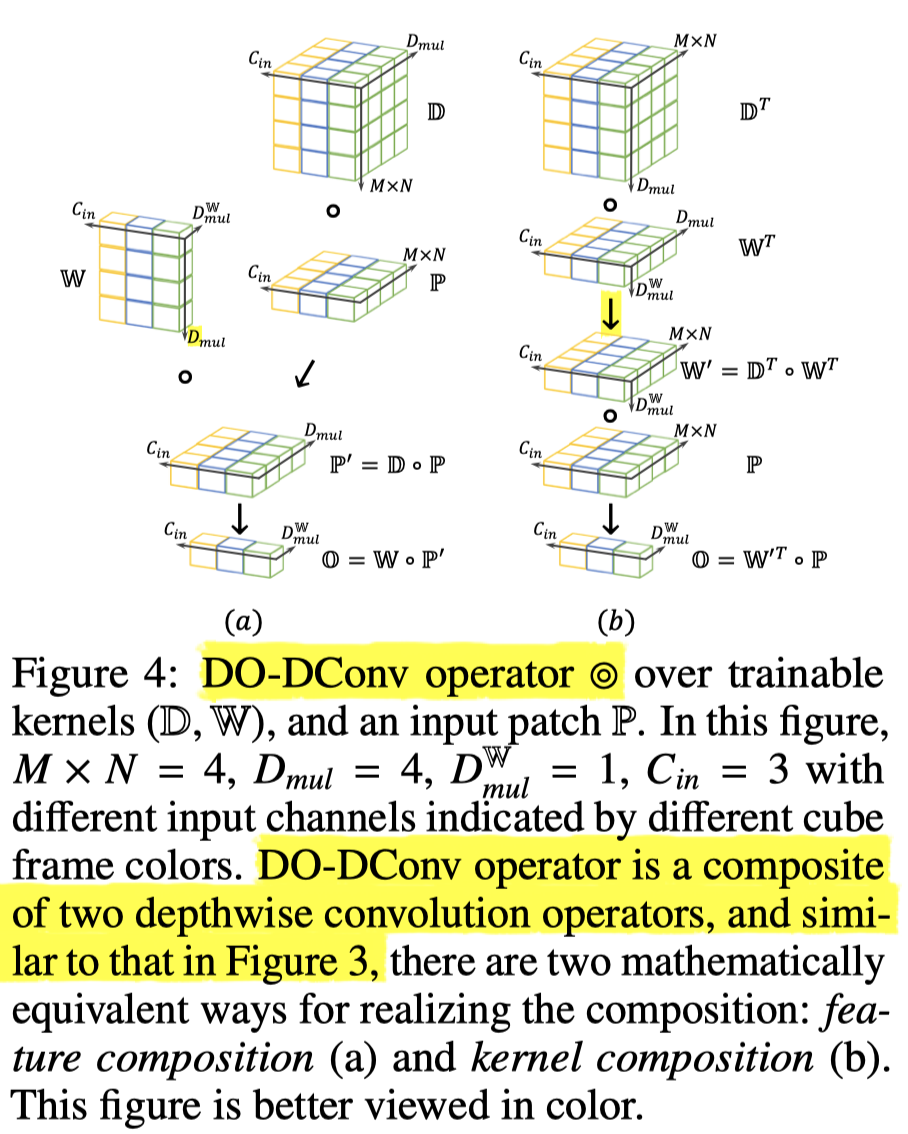### 5. Experiments

• 只贴出了分割实验的结果，其余实验详见论文；在语义分割的实验表现如下所示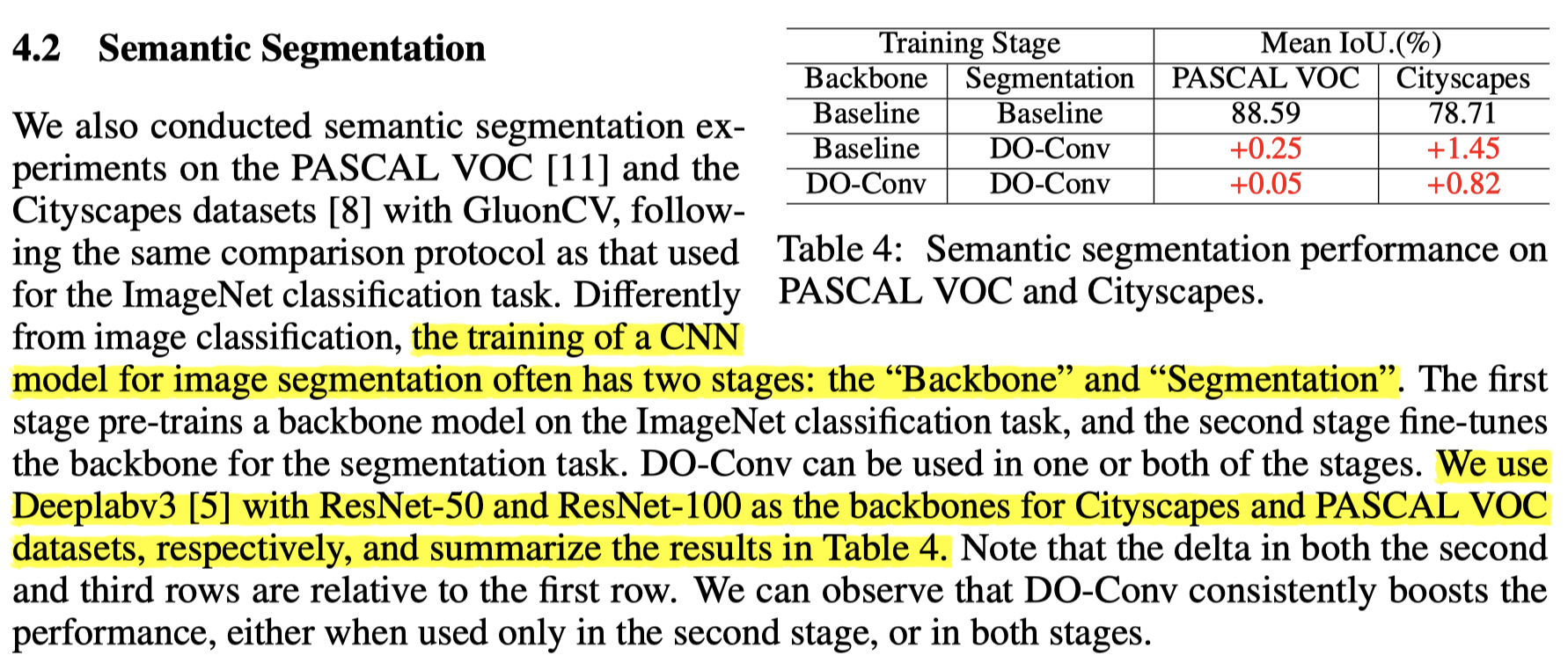### 6. Conclusions and Future work

DO-Conv, a depthwise over-parameterized convolutional layer, is a novel, simple and generic way for boosting the performance of CNNs. Beyond the practical implications of improving training and ﬁnal accuracy for existing CNNs, without introducing extra computation at the inference phase, we envision that the unveiling of its advantages could also encourage further exploration of overparameterization as a novel dimension in network architecture design.

In the future, it would be intriguing to get a theoretical understanding of this rather simple means in achieving the surprisingly non-trivial performance improvements on a board range of applications. Furthermore, we would like to expand the scope of applications where these over-parameterized convolution layers may be eﬀective, and learn what hyper-parameters can beneﬁt more from it.

-------------The End-------------